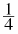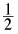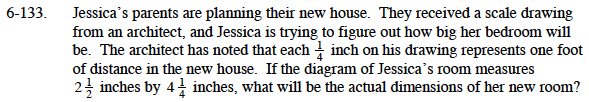### Home > MC1 > Chapter 6 > Lesson 6.3.2 > Problem6-133

6-133.

. Jessica's parents are planning their new house. They received a scale drawing from an architect, and Jessica is trying to figure out how big her bedroom will be. The architect has noted that eachinch on his drawing represents one foot of distance in the new house. If the diagram of Jessica's room measures 2inches by 4inches, what will be the actual dimensions of her new room? Homework Help ✎Change the mixed number into an improper fraction. Then change the fraction so it has a common denominator of 4. What Giant One can you use to do that?

$2\frac{1}{2}=\frac{5}{2}\;\;\;\;\;\;\;$

$\frac{5}{2}\left(\frac{2}{2}\right)=\frac{10}{4}$

Multiply the new fraction by the conversion factor to determine the number of feet. It might be helpful to think of ten-fourths as ten times one-fourth.

$10\left(\frac{1}{4}\right)\cdot \frac{\frac{1}{4}\text{ inch}}{1\text{ foot}} = 10\text{ feet}$

Now do the same thing with the other dimension to determine the length of Jessica's room.

10 feet by 17 feet